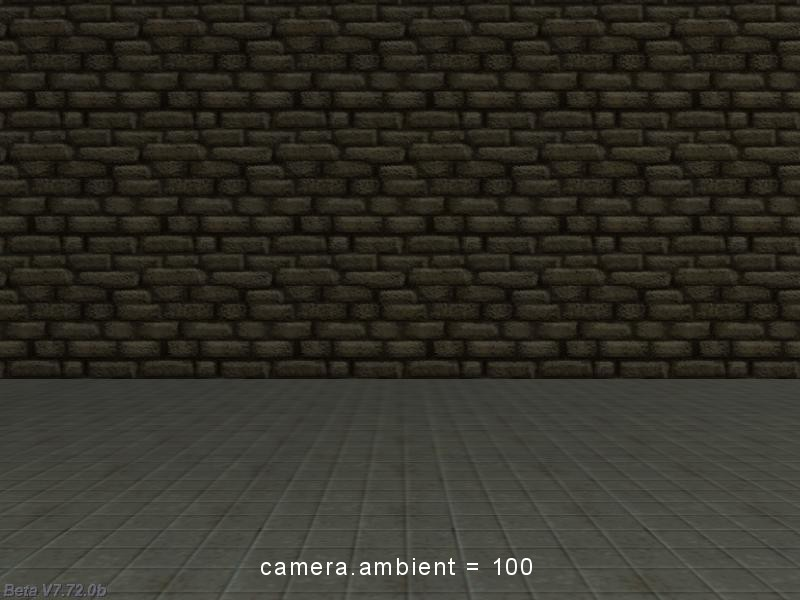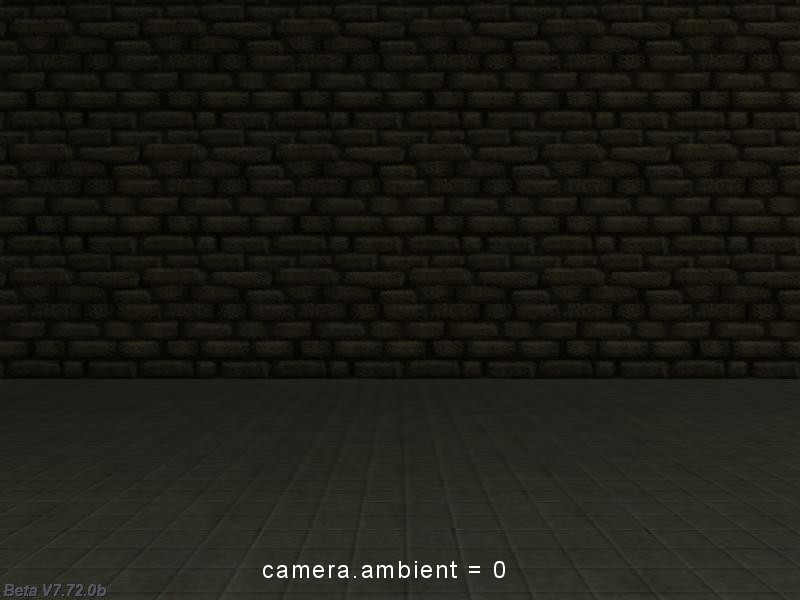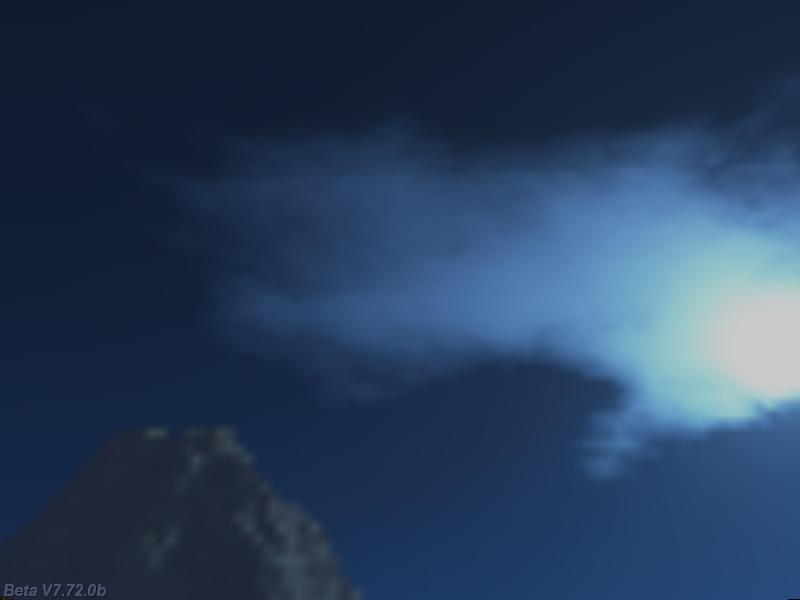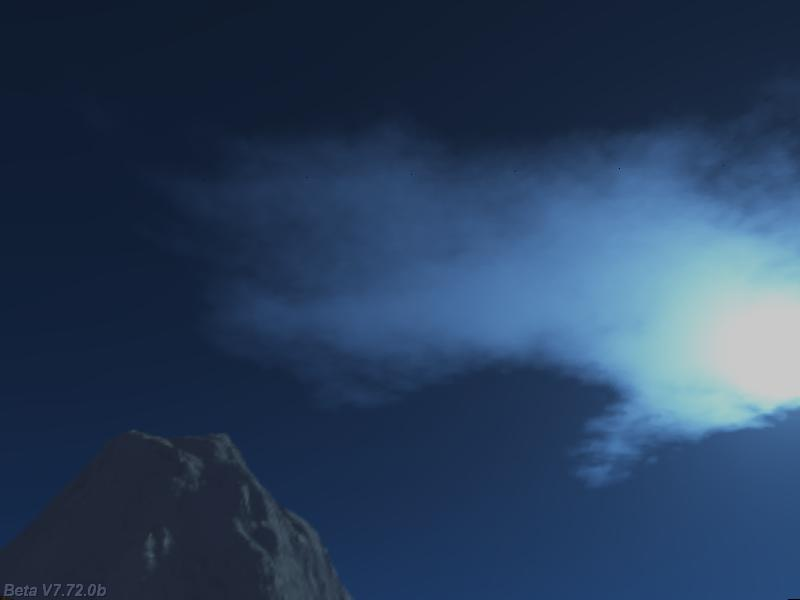Questions from the forum
 Q: I want to create a crosshair who will rotate the camera and also move around the center of the screen as I move the mouse around. A: Simply set mouse_mode to 1 to get the desired result; here's an example.   BMAP* pointer_tga = "pointer.tga";   action players_code() // attach this action to your player {        var movement_speed = 10; // movement speed        VECTOR temp;        player = my; // I'm the player        set (my, INVISIBLE); // 1st person player        while (1)        {                my.pan -= 7 * mouse_force.x * time_step;                camera.x = my.x;                camera.y = my.y;                camera.z = my.z + 50 + 1.1 * sin(my.skill44); // play with 50 and 1.1                camera.pan = my.pan;                camera.tilt += 5 * mouse_force.y * time_step;                vec_set (temp.x, my.x); // trace 10,000 quants below the player                temp.z -= 10000;                temp.z = -c_trace (my.x, temp.x, IGNORE_ME | IGNORE_PASSABLE | USE_BOX) + 15; // play with 15                temp.x = movement_speed * (key_w - key_s) * time_step;                temp.y = movement_speed * (key_a - key_d) * 0.6 * time_step;                c_move (my, temp.x, nullvector, IGNORE_PASSABLE | GLIDE);                wait (1);        } }   function mouse_startup() {         mouse_mode = 1;        mouse_map = pointer_tga;        while (1)        {                 vec_set(mouse_pos, mouse_cursor);                wait(1);        } }     Q: Assuming you had a wmb file (a simple level) with lights in it, is it possible to programmatically change the light in the level in order to make it darker or dimmer? A: Use camera.ambient to achieve what you need; here's a snippet that changes the ambient light in a loop.   function lights_startup() {        fps_max = 100;        while (1)        {                // camera.ambient will range from 0 to 100                camera.ambient = 50 + 50 * sin(3 * total_ticks); // 3 gives the speed                wait (1);        } }Q: I want my character to play its "attack" animation whenever I press the left mouse button. How can I play the entire "attack" animation by clicking my left mouse button only once? A: Use this example as a base for your code.   action player1() {        VECTOR temp;        var camera_distance = 200;        player = my; // I'm the player        while (1)        {                // player's pan is controlled by the mouse and the "A" and "D" keys                player.pan -= 10 * mouse_force.x * time_step - 1.5 * (key_a - key_d);                camera.x = player.x - camera_distance * cos(player.pan); // keep the camera behind the player                camera.y = player.y - camera_distance * sin(player.pan); // at the distance given by camera_distance                camera.z = player.z + 100; // and 100 quants above the player                camera.pan = player.pan; // the camera has the same pan angle with the player                camera.tilt += 7 * mouse_force.y * time_step; // and can tilt freely                if (!mouse_left) // the left mouse button wasn't pressed? Then take care of the regular animations (stand, walk)                {                        if (key_w + key_s > 0) // if the player is walking                        {                                ent_animate(my, "walk", my.skill46, ANM_CYCLE); // play its "walk" frames animation                                // the animation speed increases when the player presses the "shift" key                                my.skill46 += 5 * (1 + key_shift * 0.7) * time_step;                                my.skill46 %= 100; // loop the animation                        }                        else // if the player is standing                        {                                ent_animate(my, "stand", my.skill48, ANM_CYCLE); // play the "stand" frames animation                                my.skill48 += 2 * time_step; // "stand" animation speed                                my.skill48 %= 100; // loop the animation                        }                }                else // the left mouse button was pressed here                {                        my.skill40 = 0;                        while (my.skill40 < 100) // play the "attack" animation until its end                        {                                ent_animate(my, "attack", my.skill40, NULL); // play the "attack" animation only once                                my.skill40 += 2 * time_step; // "attack" animation speed                                wait (1);                        }                }                vec_set (temp, my.x); // trace 10,000 quants below the player                temp.z -= 10000;                // and adjust its height accordingly, placing its feet on the ground                temp.z = - c_trace (my.x, temp, IGNORE_ME | IGNORE_PASSABLE | USE_BOX) + 15; // play with 15                // move the player using the "W" and "S" keys; the speed increases to 200% if the player presses the "shift" key                temp.x = 10 * (key_w - key_s) * (1 + 1 * key_shift) * time_step;                temp.y = 0;                my.skill47 += c_move (my, temp, nullvector, IGNORE_PASSABLE);                wait (1);        } }     Q: What is the key mapping for double click? Is there any key mapping for this? A: There isn't any key mapping for double click; however, you can track double clicks like this:   #define click_rate 0.7 // register a double click if the player presses the left mouse button twice in less than 0.7 seconds   STRING* result_str = "#10";   TEXT* messages_txt = {        pos_x = 200;        pos_y = 20;        string(result_str);        flags = SHOW; }   function track_clicks_startup() {        var time_passed;        var doubleclick_on = 0;        while (1)        {                if (mouse_left) // the left mouse button was clicked at least once?                {                        while (mouse_left) {wait (1);} // wait until the player releases the mouse button                        time_passed = 0;                        while (time_passed < click_rate)                        {                                if (mouse_left)                                {                                        str_cpy (result_str, "Double click!");                                        doubleclick_on = 1;                                        // do what you want with the double clicks here                                }                                time_passed += time_step / 16;                                wait (1);                        }                        if (doubleclick_on == 0)                        {                                str_cpy (result_str, "Single click!");                                // do what you want with the single clicks here                                time_passed = 0;                                while (time_passed < 0.5) // display the "Single click!" message for 0.5 seconds                                {                                        time_passed += time_step / 16;                                        wait (1);                                }                                }                }                else // the left mouse button wasn't clicked at all here                {                        doubleclick_on = 0;                        str_cpy (result_str, "No mouse clicks!");                }                wait (1);        } }     Q: Imagine a plane flying towards a destination that is out of view; I need a needle to point towards the destination. Can you help? A: Use the code below.   ENTITY* plane_target;   action my_target() {        plane_target = my; }   function my_arrow() {        set (my, PASSABLE);        my.ambient = 100;        VECTOR arrow_coords;        VECTOR temp;        while (1)        {                // place the arrow at these xyz coordinates in relation to the player                vec_set(arrow_coords.x, vector(300, -100, 0));                vec_rotate(arrow_coords.x, player.pan);                vec_add(arrow_coords, player.x);                vec_set(my.x, arrow_coords.x);                vec_set(temp, plane_target.x);                vec_sub(temp, my.x);                vec_to_angle(my.pan, temp);                // my.tilt = 0; // remove the comment if you want to keep the arrow movement in plain 2D                wait (1);        } }   action my_plane() // just a poor sample {        VECTOR temp;        player = my;        while (!plane_target) {wait (1);}        ent_create ("arrow.mdl", nullvector, my_arrow);        while (1)        {                my.pan -= 7 * mouse_force.x * time_step;                camera.x = my.x;                camera.y = my.y;                camera.z = my.z;                camera.pan = my.pan;                camera.tilt += 5 * mouse_force.y * time_step;                temp.x = 10 * (key_w - key_s) * time_step;                temp.y = 5 * (key_a - key_d) * 0.6 * time_step;                temp.z = 0;                c_move (my, temp.x, nullvector, IGNORE_PASSABLE | GLIDE);                wait (1);        } }     Q: Is there a way to scale my sky box texture (skybox+6.tga) so it doesn't get so stretched when it is created? A: The solution is simple: use a texture with a big resolution. Compare the shots below; the first one uses a 768 x 128 pixels bitmap, while the second one uses a 3072 x 512 pixels bitmap.Q: Is it possible to have collision detection between a hmp terrain and snow particles? A: The big difference between sprites and particles is speed; this is achieved by ignoring the collision detection for the particles. Nevertheless, you can fake the collisions in a convincing way by playing with the lifespan for your particles, making sure that they die right after they penetrate the terrain.   BMAP* snow_tga = "snow.tga";   function snow_effect(PARTICLE *p) {        p->vel_x = 1 - random(2);        p->vel_y = 1 - random(2);        p->vel_z = -5 + random(3);        p.lifespan = 70; // this value sets the lifespan for your particles - play with it        p.alpha = 40 + random(50);        p.bmap = snow_tga;        p.size = 3;        p.flags |= (BRIGHT | MOVE);        p.event = NULL; }   action snow_generator() // place a model above the terrain and attach it this action {        VECTOR temp;        set (my, INVISIBLE | PASSABLE);        while (1)        {                temp.x = my.x + 1000 - random(2000); // generate snow particles in a range of -1000...+1000 quants                temp.y = my.y + 1000 - random(2000); // around the object on the x and y axis                temp.z = my.z;                effect(snow_effect, 1, temp.x, nullvector);                temp.x = my.x + 1000 - random(2000);                temp.y = my.y + 1000 - random(2000);                temp.z = my.z;                effect(snow_effect, 1, temp.x, nullvector);                temp.x = my.x + 1000 - random(2000);                temp.y = my.y + 1000 - random(2000);                temp.z = my.z;                effect(snow_effect, 1, temp.x, nullvector);                temp.x = my.x + 1000 - random(2000);                temp.y = my.y + 1000 - random(2000);                temp.z = my.z;                effect(snow_effect, 1, temp.x, nullvector);                temp.x = my.x + 1000 - random(2000);                temp.y = my.y + 1000 - random(2000);                temp.z = my.z;                effect(snow_effect, 1, temp.x, nullvector); // generate 5 snow particles each frame                wait (1);        } }     Q: I want to make a particle that can rotate together with the entity that it is attached to. A: Setting a small lifespan value for your particles will destroy them right after their creation. This way,  you can achieve what you want by simply generating a new particle each frame.   BMAP* effect_tga = "snow.tga";   function my_effect(PARTICLE *p) {        p.lifespan = 1; // kill the particle right after its creation        p.alpha = 50;        p.bmap = effect_tga;        p.size = 5;        p.flags |= (BRIGHT | MOVE);        p.event = NULL; }   action rotating_object() // just an example {        VECTOR temp;        while (1)        {                my.pan += 3 * time_step;                my.tilt += 4 * time_step;                my.roll += 2 * time_step;                vec_for_vertex(temp, my, 10); // generate particles from the 10th vertex of the entity                effect(my_effect, 1, temp.x, nullvector);                wait (1);        } }     Q: I have a entity that walks onto a path but I want him to move off that path when the player comes into his scan zone. A: Use the code below.   var entity_speed = 3; var movement_enabled = 0; var dist_to_node; var current_node = 1; var angle_difference = 0;   VECTOR temp_angle; VECTOR pos_node; // stores the position of the node   function move_target() {        while(1)        {                if(movement_enabled)                {                        entity_speed = minv(5, entity_speed + 0.5 * time_step);                        ent_animate(my, "walk", my.skill46, ANM_CYCLE); // play its "walk" frames animation                        my.skill46 += 5 * time_step;                        my.skill46 %= 100; // loop the animation                        c_move(my, vector(entity_speed * time_step, 0, 0), nullvector, IGNORE_PASSABLE | GLIDE);                        vec_to_angle (my.pan, vec_diff (temp_angle, pos_node, my.x));                }                wait(1);        } }   action my_enemy() // attach this action to your enemy model {         VECTOR temp;        while (!player) {wait (1);}             move_target();        result = path_scan(me, my.x, my.pan, vector(90, 80, 400)); // scan the area looking for the player        if (result) {movement_enabled = 1;}        path_getnode (my, 1, pos_node, NULL);        vec_to_angle (my.pan, vec_diff (temp_angle, pos_node, my.x)); // rotate towards the node        while(1)        {                if ((c_scan(my.x, my.pan, vector(180, 90, 1000), IGNORE_ME) > 0) && (you == player)) // detected the player?                        break; // then get out of this loop!                dist_to_node = vec_dist(my.x, pos_node);                if(dist_to_node < 50) // close to the node?                {                        current_node = path_nextnode(my, current_node, 1);                        if (!current_node) {current_node = 1;} // reached the end of the path? Then start over!                        path_getnode (my, current_node, pos_node, NULL);                }                wait(1);        }        // the enemy has spotted the player here        while (1) // so it rotates towards it and starts chasing it        {                vec_set(temp, player.x);                vec_sub(temp, my.x);                vec_to_angle(my.pan, temp);                my.tilt = 0;                wait (1);        } }     Q: I'm not sure what about the numerical scancode values that are returned on my PC. How can I check the values for my joystick? A: Here's a simple project that returns the values for the keys, mouse buttons, joysticks, etc.   void main() {        video_screen = 1; // start in full screen mode        level_load (test_wmb);        fps_max = 70;        video_mode = 7; // run in 800x600 pixels        video_depth = 32; // 32 bit mode        while (1)        {                while (!key_any) {wait (1);} // wait until a key is pressed                while (key_any) {wait (1);} // wait until a key is released                wait (1);        } }   PANEL* scancodes_pan = {        digits = 30, 50, 5, *, 1, key_lastpressed; // display the scan code for the last key that was pressed        flags = SHOW; }